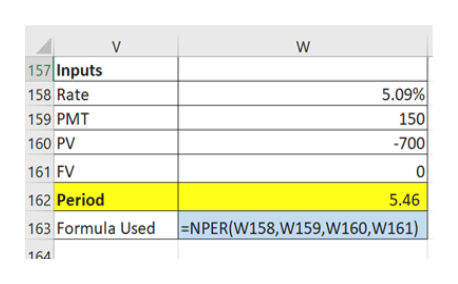# You borrowed \$700 at 5% compounded quarterly. Yourpayments are \$150 at the end of each year. How many years will you make payments on the loan?(Hint: compounding frequency is different from payment frequency; we know that r and t should be matched; compounding effect? Effective interest rate?)

Question
50 views

You borrowed \$700 at 5% compounded quarterly. Your
payments are \$150 at the end of each year. How many years will you make payments on the loan?

(Hint: compounding frequency is different from payment frequency; we know that r and t should be matched; compounding effect? Effective interest rate?)

check_circle

Step 1

We will adopt the following approach:

• It's a typical time value of money case, where we have to find nper i.e. net period the loan will remain outstanding under annual payment.
• However, there is one small catch. he payment frequency is annual. Hence the period here is 1 year. However the interest is getting compounded quarterly. Henece, we need to calculate the interest rate per period i.e. interest rate per annuam i.e. the effective annual rate (EAR).
• EAR will match the interest rate and the frequency of payment to annual basis
• After that we will use the NPER function of excel to get the number of years the loan will remain outstanding.
Step 2

Inputs of NPER function:

PV of loan = - Borrwed amount = - \$ 700

Rate = EAR = (1 + 5% / 4)4 - 1 = 5.09%

PMT = Payment per period = \$ 150

FV = Future value = 0

Step 3

Please see the table on the white board for calculation of total period of loan using NPER function of excel.The last row highlighted in yel...help_outlineImage TranscriptioncloseV 157 Inputs 5.09% 158 Rate 150 700 159 PMT 160 PV 161 FV 0 162 Period 5.46 163 Formula Used =NPER(W158,W159,W160,W161) 164 fullscreen

### Want to see the full answer?

See Solution

#### Want to see this answer and more?

Solutions are written by subject experts who are available 24/7. Questions are typically answered within 1 hour.*

See Solution
*Response times may vary by subject and question.
Tagged in